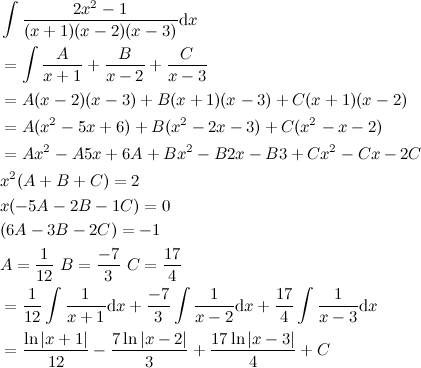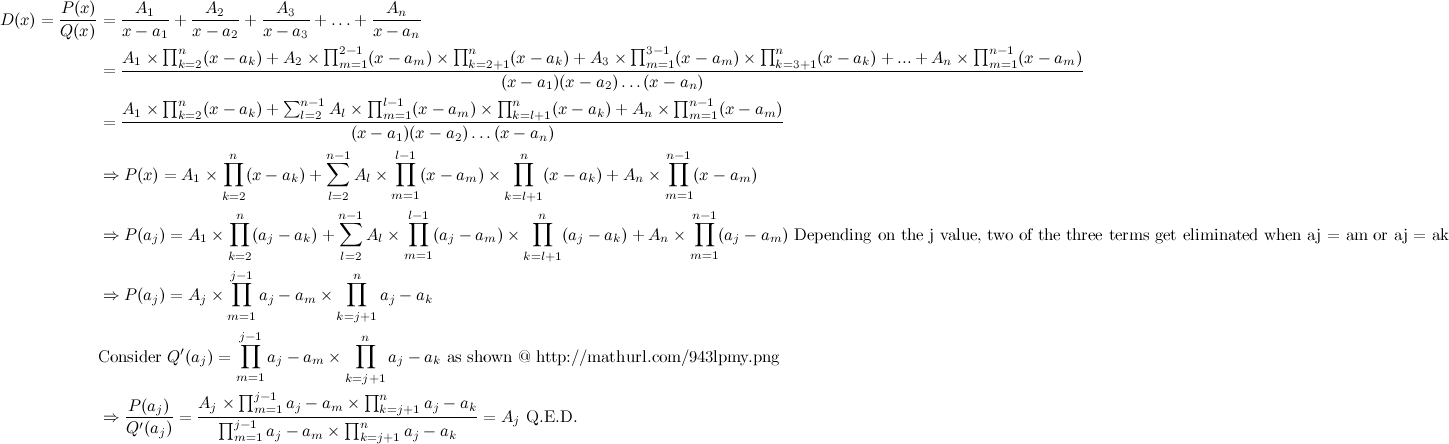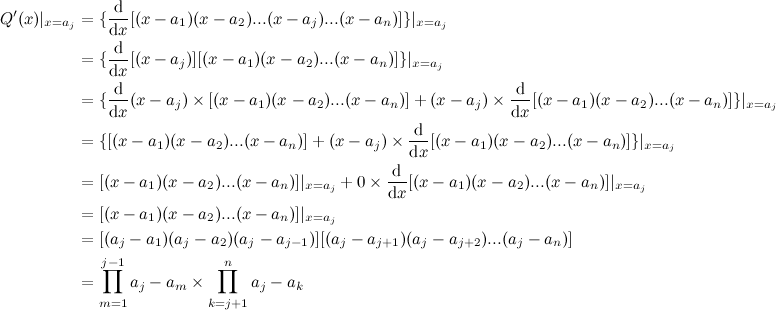# Thread: Wait, wah...? Partial Fractions and Derivative Proof, I think..?

1. ##Wait, wah...? Partial Fractions and Derivative Proof, I think..?

72a. I have no frigging clue where to start.Reply With Quote

2. ##Re: Wait, wah...? Partial Fractions and Derivative Proof, I think..?

isn't this a Riemann summation? It's been a longtime since I've done that, but it looks really similar.Reply With Quote

3. ##Re: Wait, wah...? Partial Fractions and Derivative Proof, I think..?

Just bumping this post up to say no. @Locked, you have any ideas?Reply With Quote

4. ##Re: Wait, wah...? Partial Fractions and Derivative Proof, I think..?

Where's @Noah when you need him?Reply With Quote

5. ##Re: Wait, wah...? Partial Fractions and Derivative Proof, I think..?

Other than it being a seemingly useless proof, not really.My friend proved it though. I'll tell him to post here.

By useless, I mean that it doesn't make anything easier really. You could do the second problem (72b) without proving anything and just using regular partial fractions. Though that ruins the spirit of the problem ;pReply With Quote

6. ##Re: Wait, wah...? Partial Fractions and Derivative Proof, I think..?

Full proof:Basically just do the same thing you do for a normal partial fraction problem, just with 10x more symbols and a little bit of reasoning. There's a sketchy part in expressing the product from m=1 to j-1 when j can very well be 1. I can easily do a piece-wise solution (when j = 1, when j = n, and otherwise), but you get the idea of the proof anyway. And by "two of the three terms", I mean either the A1 term remains, or the An term remains, or Aj remains where 1 < j < n. Better wording should say "all but one term get eliminated" instead.

EDIT: Long time no see, guys.Reply With Quote

7. ##Re: Wait, wah...? Partial Fractions and Derivative Proof, I think..?

Holy sh`it he hasn't posted since 2010.

It's been that long?Reply With Quote

8. ##Re: Wait, wah...? Partial Fractions and Derivative Proof, I think..?

0.o im in 10th grade and i think my brain just exploded. THIS IS MATH ISNT IT?? THIER ARE NO NUMBERS??Reply With Quote

9. ##Re: Wait, wah...? Partial Fractions and Derivative Proof, I think..?

Math stops having a lot of numbers at around the Calculus level and is replaced with symbols.Reply With Quote

10. ##Re: Wait, wah...? Partial Fractions and Derivative Proof, I think..?

great... just when i was starting to understand math.Reply With Quote

11. ##Re: Wait, wah...? Partial Fractions and Derivative Proof, I think..?

Symbols represent numbers.Reply With Quote

12. ##Re: Wait, wah...? Partial Fractions and Derivative Proof, I think..?

Here's an English translation:

- Step 1: Clear the denominator by (1) multiplying each numerator (A1, A2, ..., Aj, ..., An) with every (x - aj) except for the one directly below it and (2) summing them together.
- Step 2: Notice that each term of this pattern: Aj * (x - a[everything else except j]), thus the only term that is left when plugging in x = aj is the one that does not have (x - aj) in it. Incidentally, this is the Aj term.
- Step 3: So P(aj) = Aj * (aj - a1) * (aj - a2) * ... * (aj - aj-1) * (aj - aj+1) * ... * (aj - an)
- Step 4: Take the derivative of Q(x), using product rule after re-ordering Q(x) into (x - aj) * everything else. Q'(x) = (x - aj)' * everything else + (x - aj) * (everything else)'
- Step 5: The second part is 0 when plugging in x = aj, so Q'(aj) = everything else = (aj - a1) * (aj - a2) * ... * (aj - aj-1) * (aj - aj+1) * ... * (aj - an)
- Step 6: P(aj)/Q'(aj) = AjReply With Quote

13. ##Re: Wait, wah...? Partial Fractions and Derivative Proof, I think..?

I can't see the page for some reason, but if you were trying to isolate Q'(x) I think the 2nd method is a better proof, academically speaking. Also in terms of clarity.Reply With Quote

14. ##Re: Wait, wah...? Partial Fractions and Derivative Proof, I think..?

The second one is a proof of a statement in the first one.Reply With Quote

15. ##Re: Wait, wah...? Partial Fractions and Derivative Proof, I think..?

Ah ok I see why that was necessary now.Reply With Quote

16. ##Re: Wait, wah...? Partial Fractions and Derivative Proof, I think..?

Bro, I am in calculus and I had to spend like half an hour trying to process this.

Thanks Kalovale.Reply With Quote

17. ##Re: Wait, wah...? Partial Fractions and Derivative Proof, I think..?

I feel like we need more math questions in here.Reply With Quote

18. ##Re: Wait, wah...? Partial Fractions and Derivative Proof, I think..?

I don't have that fancy math-writing program, sorry if it's sloppy :(

f(x,y) = xy + 64/x + 64/y

Local Maximum values(s) : DNE [correct]

Local Minimum values(s) : 48 Fixed it; works now

Saddle points(s) (x, y, f) = DNE [correct]

I think I'm putting the answer in the wrong form..?

First derivatives:
fx = y - 64/x^2; fy = x - 64/y^2

Second derivatives:
fxx = 128/x^3; fyy = 128/y^3; fxy = 1

Set first derivatives = 0, substitute:
y = 64/x^2; x = 64/y^2
x = 64/(64/x^2)^2 = x^4/64

Solve for x:
x(x^3 - 64) = 0
x = 0, x = 4

Function is undefined at x = 0.

Substituting x = 4 into fx gives y = 4.

D(x,y) = fxx(x,y)*fyy(x,y) - fxy(x,y)
D(4,4) = (2)(2) - 1 = 3

Since D(4,4) > 0 and fxx(4,4) > 0, f(4,4) is a local minimum. Therefore, there are no local maximum or saddle points.

WebAssign is not accepting (4,4) as an answer. I have tried (4,4,48) [for (x,y,f) format?], f(4,4), f(4,4)=48. Is there a standard form for answering that I missed?

EDIT: 48 worked. Guess value(s) should have given it away, since it wasn't asking for a point.

I'll probably be looking for more math help next semester and post a few questions.Reply With Quote

19. ##Re: Wait, wah...? Partial Fractions and Derivative Proof, I think..?

It ain't fancy. It's just LaTeX.Reply With Quote

####Posting Permissions

• You may not post new threads
• You may not post replies
• You may not post attachments
• You may not edit your posts
•